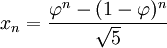The Golden Ratio to Calculate Fibonacci Numbers

Recommended Posts

Hello, everyone

This is my first forum. I need help to find the nth term of Fibonacci series with the golden ratio. Can anyone help me to know about golden ratio and its equation? and how to find Fibonacci series from it.

Share on other sites

Do you know about the golden ratio?

Share on other sites

Golden ratio - Wikipedia

In mathematics, two quantities are in the golden ratio if their ratio is the same as the ratio of their sum to the larger of the two quantities. ... where the Greek letter phi ( φ {\displaystyle \varphi } or ϕ {\displaystyle \phi } ) represents the golden ratio. Its value is: φ = 1 + 5 2 = 1.6180339887 … .

Decimal‎: ‎1.6180339887498948482... A...
Binary‎: ‎1.1001111000110111011
Share on other sites

Definition: The golden ratio, also known as the divine proportion, golden mean, or golden section, is a number often encountered when taking the ratios of distances in simple geometric figures such as the pentagon, pentagram, decagon and dodecahedron. It is denoted, or sometimes.

Using The Golden Ratio to Calculate Fibonacci Numbers

The Golden Ratio to calculate Fibonacci Series:The answer will be equal to the addition of the previous two terms, as a whole number:

I.E.:When I used an online calculator for this, I only enter the Golden Ratio to 6 decimal places & I got the answer 8.00000033. A more accurate calculation would be closer to 8.

Edited by AshBox
Share on other sites

Golden ratio - Wikipedia

In mathematics, two quantities are in the golden ratio if their ratio is the same as the ratio of their sum to the larger of the two quantities. ... where the Greek letter phi ( φ {\displaystyle \varphi } or ϕ {\displaystyle \phi } ) represents the golden ratio. Its value is: φ = 1 + 5 2 = 1.6180339887 … .

Decimal‎: ‎1.6180339887498948482... A...
Binary‎: ‎1.1001111000110111011

Thank you for great information.

Definition: The golden ratio, also known as the divine proportion, golden mean, or golden section, is a number often encountered when taking the ratios of distances in simple geometric figures such as the pentagon, pentagram, decagon and dodecahedron. It is denoted, or sometimes.

Using The Golden Ratio to Calculate Fibonacci Numbers

The Golden Ratio to calculate Fibonacci Series:The answer will be equal to the addition of the previous two terms, as a whole number:

I.E.:When I used an online calculator for this, I only enter the Golden Ratio to 6 decimal places & I got the answer 8.00000033. A more accurate calculation would be closer to 8.

Thank You AshBox. I have tried this equation and it gave me exact result which I was wondering about.

Create an account

Register a new account